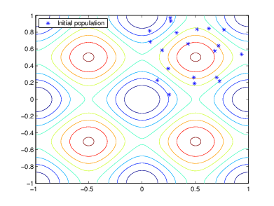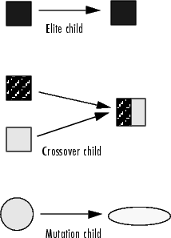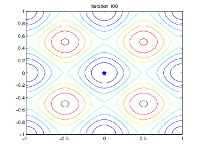Main Content

## How the Genetic Algorithm Works

### Outline of the Algorithm

The following outline summarizes how the genetic algorithm works:

1. The algorithm begins by creating a random initial population.

2. The algorithm then creates a sequence of new populations. At each step, the algorithm uses the individuals in the current generation to create the next population. To create the new population, the algorithm performs the following steps:

1. Scores each member of the current population by computing its fitness value. These values are called the raw fitness scores.

2. Scales the raw fitness scores to convert them into a more usable range of values. These scaled values are called expectation values.

3. Selects members, called parents, based on their expectation.

4. Some of the individuals in the current population that have lower fitness are chosen as elite. These elite individuals are passed to the next population.

5. Produces children from the parents. Children are produced either by making random changes to a single parent—mutation—or by combining the vector entries of a pair of parents—crossover.

6. Replaces the current population with the children to form the next generation.

3. The algorithm stops when one of the stopping criteria is met. See Stopping Conditions for the Algorithm.

### Initial Population

The algorithm begins by creating a random initial population, as shown in the following figure.In this example, the initial population contains `20` individuals. Note that all the individuals in the initial population lie in the upper-right quadrant of the picture, that is, their coordinates lie between 0 and 1. For this example, the `InitialPopulationRange` option is `[0;1]`.

If you know approximately where the minimal point for a function lies, you should set `InitialPopulationRange` so that the point lies near the middle of that range. For example, if you believe that the minimal point for Rastrigin's function is near the point `[0 0]`, you could set `InitialPopulationRange` to be `[-1;1]`. However, as this example shows, the genetic algorithm can find the minimum even with a less than optimal choice for `InitialPopulationRange`.

### Creating the Next Generation

At each step, the genetic algorithm uses the current population to create the children that make up the next generation. The algorithm selects a group of individuals in the current population, called parents, who contribute their genes—the entries of their vectors—to their children. The algorithm usually selects individuals that have better fitness values as parents. You can specify the function that the algorithm uses to select the parents in the `SelectionFcn` option. See Selection Options.

The genetic algorithm creates three types of children for the next generation:

• Eliteare the individuals in the current generation with the best fitness values. These individuals automatically survive to the next generation.

• Crossover are created by combining the vectors of a pair of parents.

• Mutation children are created by introducing random changes, or mutations, to a single parent.

The following schematic diagram illustrates the three types of children.Mutation and Crossover explains how to specify the number of children of each type that the algorithm generates and the functions it uses to perform crossover and mutation.

The following sections explain how the algorithm creates crossover and mutation children.

#### Crossover Children

The algorithm creates crossover children by combining pairs of parents in the current population. At each coordinate of the child vector, the default crossover function randomly selects an entry, or gene, at the same coordinate from one of the two parents and assigns it to the child. For problems with linear constraints, the default crossover function creates the child as a random weighted average of the parents.

#### Mutation Children

The algorithm creates mutation children by randomly changing the genes of individual parents. By default, for unconstrained problems the algorithm adds a random vector from a Gaussian distribution to the parent. For bounded or linearly constrained problems, the child remains feasible.

The following figure shows the children of the initial population, that is, the population at the second generation, and indicates whether they are elite, crossover, or mutation children.### Plots of Later Generations

The following figure shows the populations at iterations 60, 80, 95, and 100.As the number of generations increases, the individuals in the population get closer together and approach the minimum point ```[0 0]```.

### Stopping Conditions for the Algorithm

The genetic algorithm uses the following options to determine when to stop. See the default values for each option by running ```opts = optimoptions('ga')```.

• `MaxGenerations` — The algorithm stops when the number of generations reaches `MaxGenerations`.

• `MaxTime` — The algorithm stops after running for an amount of time in seconds equal to `MaxTime`.

• `FitnessLimit` — The algorithm stops when the value of the fitness function for the best point in the current population is less than or equal to `FitnessLimit`.

• `MaxStallGenerations` — The algorithm stops when the average relative change in the fitness function value over `MaxStallGenerations` is less than ```Function tolerance```.

• `MaxStallTime` — The algorithm stops if there is no improvement in the objective function during an interval of time in seconds equal to `MaxStallTime`.

• `FunctionTolerance` — The algorithm runs until the average relative change in the fitness function value over `MaxStallGenerations` is less than ```Function tolerance```.

• `ConstraintTolerance` — The `ConstraintTolerance` is not used as stopping criterion. It is used to determine the feasibility with respect to nonlinear constraints. Also, `max(sqrt(eps),ConstraintTolerance)` determines feasibility with respect to linear constraints.

The algorithm stops as soon as any one of these conditions is met.

### Selection

The selection function chooses parents for the next generation based on their scaled values from the fitness scaling function. The scaled fitness values are called the expectation values. An individual can be selected more than once as a parent, in which case it contributes its genes to more than one child. The default selection option, `@selectionstochunif`, lays out a line in which each parent corresponds to a section of the line of length proportional to its scaled value. The algorithm moves along the line in steps of equal size. At each step, the algorithm allocates a parent from the section it lands on.

A more deterministic selection option is `@selectionremainder`, which performs two steps:

• In the first step, the function selects parents deterministically according to the integer part of the scaled value for each individual. For example, if an individual's scaled value is 2.3, the function selects that individual twice as a parent.

• In the second step, the selection function selects additional parents using the fractional parts of the scaled values, as in stochastic uniform selection. The function lays out a line in sections, whose lengths are proportional to the fractional part of the scaled value of the individuals, and moves along the line in equal steps to select the parents.

Note that if the fractional parts of the scaled values all equal 0, as can occur using `Top` scaling, the selection is entirely deterministic.

For details and more selection options, see Selection Options.

### Reproduction Options

Reproduction options control how the genetic algorithm creates the next generation. The options are

• `EliteCount` — The number of individuals with the best fitness values in the current generation that are guaranteed to survive to the next generation. These individuals are called elite children.

When `EliteCount` is at least 1, the best fitness value can only decrease from one generation to the next. This is what you want to happen, since the genetic algorithm minimizes the fitness function. Setting `EliteCount` to a high value causes the fittest individuals to dominate the population, which can make the search less effective.

• `CrossoverFraction` — The fraction of individuals in the next generation, other than elite children, that are created by crossover. Setting the Crossover Fraction describes how the value of `CrossoverFraction` affects the performance of the genetic algorithm.

Because elite individuals have already been evaluated, `ga` does not reevaluate the fitness function of elite individuals during reproduction. This behavior assumes that the fitness function of an individual is not random, but is a deterministic function. To change this behavior, use an output function. See `EvalElites` in The State Structure.

### Mutation and Crossover

The genetic algorithm uses the individuals in the current generation to create the children that make up the next generation. Besides elite children, which correspond to the individuals in the current generation with the best fitness values, the algorithm creates

• Crossover children by selecting vector entries, or genes, from a pair of individuals in the current generation and combines them to form a child

• Mutation children by applying random changes to a single individual in the current generation to create a child

Both processes are essential to the genetic algorithm. Crossover enables the algorithm to extract the best genes from different individuals and recombine them into potentially superior children. Mutation adds to the diversity of a population and thereby increases the likelihood that the algorithm will generate individuals with better fitness values.

See Creating the Next Generation for an example of how the genetic algorithm applies mutation and crossover.

You can specify how many of each type of children the algorithm creates as follows:

• `EliteCount` specifies the number of elite children.

• `CrossoverFraction` specifies the fraction of the population, other than elite children, that are crossover children.

For example, if the `PopulationSize` is `20`, the `EliteCount` is `2`, and the `CrossoverFraction` is `0.8`, the numbers of each type of children in the next generation are as follows:

• There are two elite children.

• There are 18 individuals other than elite children, so the algorithm rounds 0.8*18 = 14.4 to 14 to get the number of crossover children.

• The remaining four individuals, other than elite children, are mutation children.

Watch now